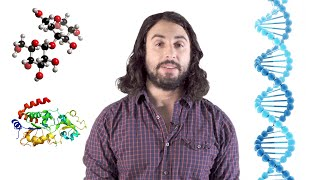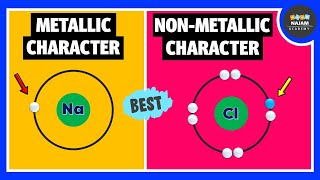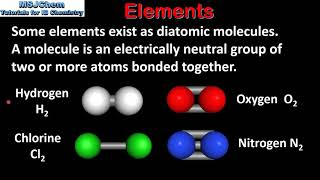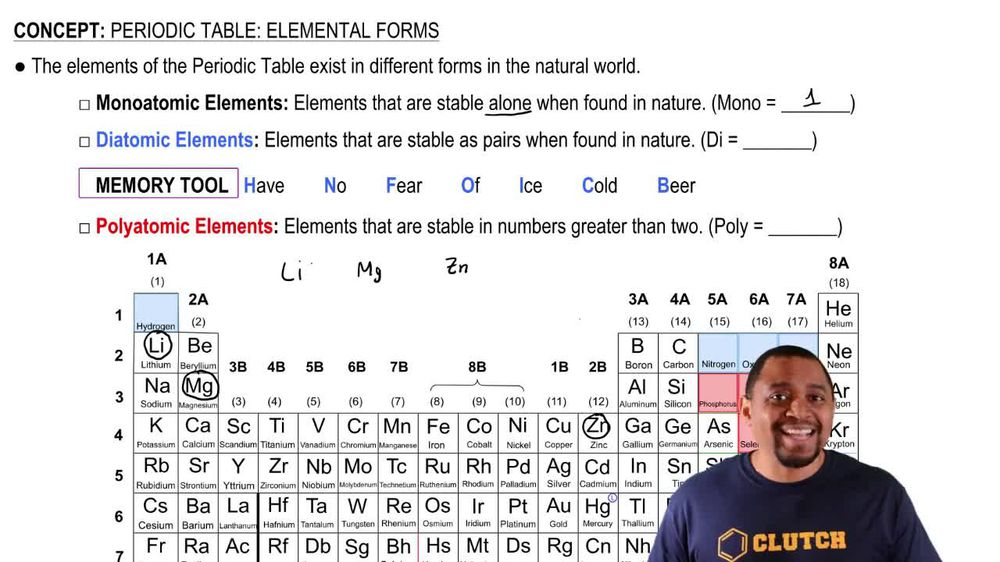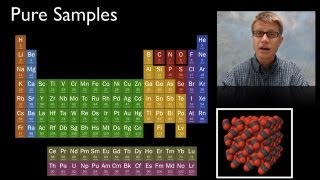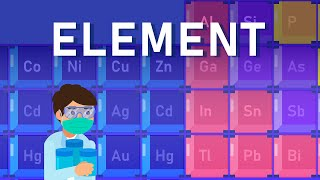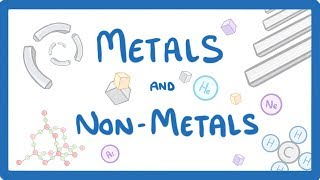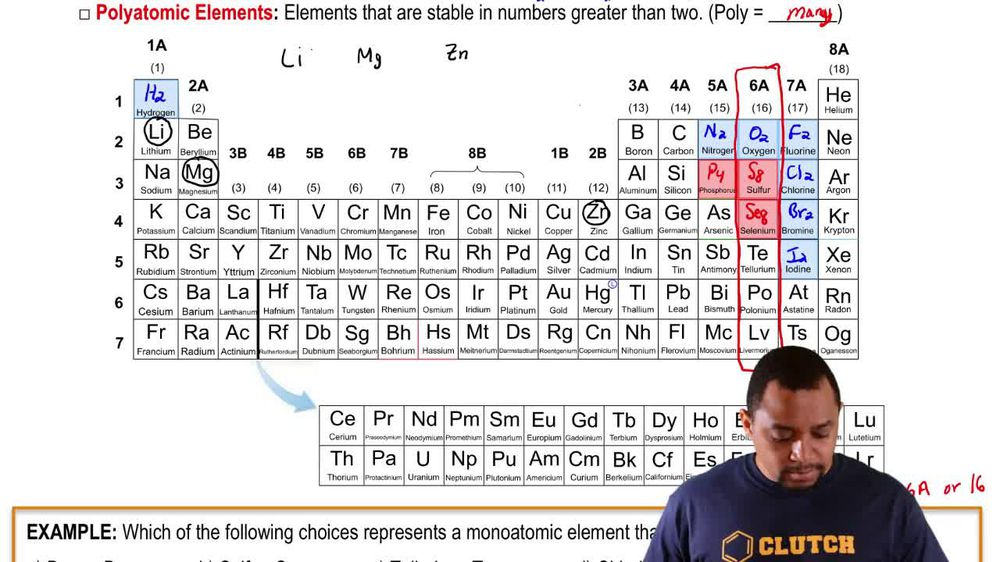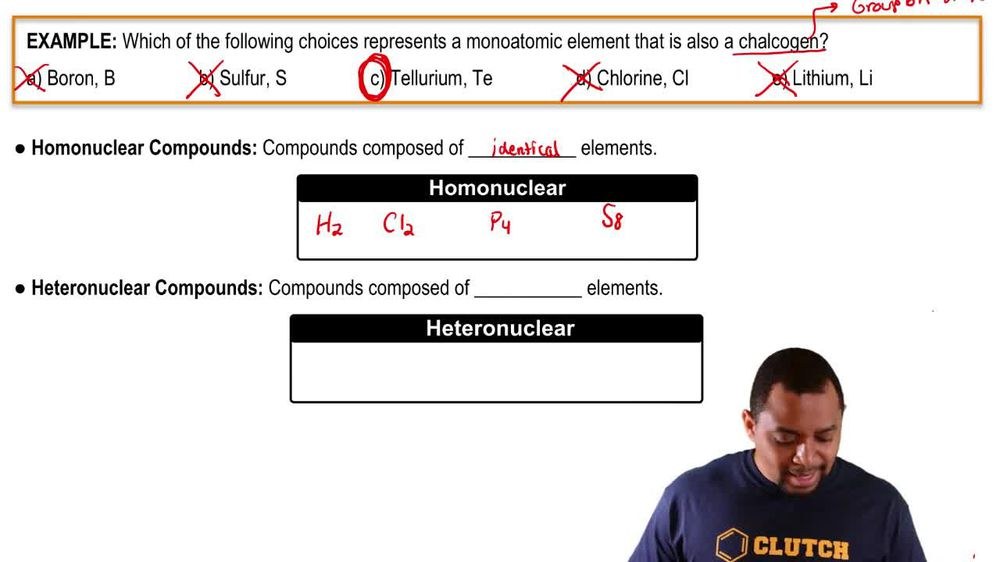Start typing, then use the up and down arrows to select an option from the list.
1. 2. Atoms & Elements2. Periodic Table: Elemental Forms
Problem

# Identify the elements that have molecules as their basic units. a. hydrogen b. iodine c. lead d. oxygen

Relevant Solution3m
Play a video:
hey everyone in this example, we're given the below elements that we need to classify as either atomic or molecular. So what we should recall is our definitions for atomic and molecular elements, we should recall that an atomic element is going to be an element that can exist as independent atoms. Whereas we want to recall molecular elements are chemical species that have at least two. Sorry to atoms of the same element bonded to each other. Okay. And so beginning with part A were given chlorine. We want to recall chlorine location on our periodic tables which we would see is located in group seven A. And so therefore we would recall Adams in Group seven A. Are in the halogen group. And so because we know that it's a halogen, we would recall halogen R. Di atomic molecules And so we would form cl two to represent our chlorine. And so therefore we can say it's going to be considered a molecular element. So this would be our our answer for part A. Moving on to Part two. Were given the atom helium, We should recall helium location on the periodic table and group eight a. And so therefore we would recall Group eight A. Represents our noble gas group on the periodic table. And so because it's a noble gas, it's going to be able to exist independently as an atom. And so therefore we can label this as atomic for part B. So this is our answer for B. Helium atomic. Moving on to nitrogen, we recall its location on our periodic table in group five a. And so therefore we would go ahead and recognize this as a di atomic gas Because it's in group five a. And so because it's a di atomic guess we can go ahead and say that this is going to be molecular because we would for men too. So this would be our answer for part C. Of this question. And lastly we have part D. When we recall the location for potassium on our periodic tables, we see that it's located in group one A. And so therefore we should recall Group one A. Elements are alkali metals. And so that means that it can exist on its own as an independent atom. And so it would be labeled as atomic because we should recall that all medals our atomic and so this would be our final answer to complete part D. And this entire example for this question. So I hope that everything be reviewed is clear. If you have any questions, please leave them down below and I will see everyone in the next practice video